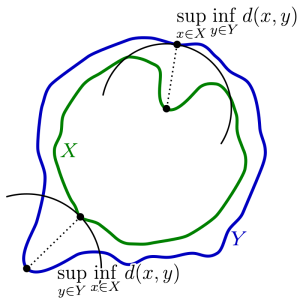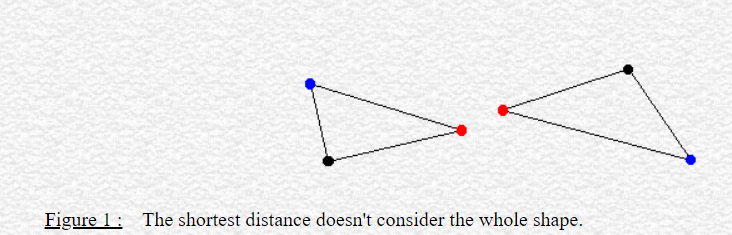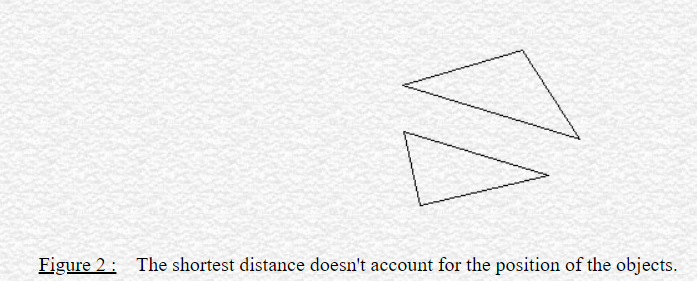Categories

## Finding the Lower Bounds of the Hausdorff Distance

Author: Bryce Van Ross

Currently, we’re finishing the first half of the 2-week Robust computation of the Hausdorff distance between triangle meshes project. This research is lead by mentor Dr. Leonardo Sacht, TA Erik Amézquita, and in my immediate team, I work with fellow students Deniz Ozbay and Talant Talipov. The below is a brief summary of  what’s happened so far:

A mathematical visualization of the Hausdorff distance of two meshesApplications: Primarily computer vision, computer graphics, digital fabrication, 3D-printing, and modeling. For example, in computer vision, it is often desirable to identify a best-candidate target relative to some initial template. In reference to the set of points within the template, the Hausdorff distance can be computed for  each potential target. The target with the minimum Hausdorff distance would qualify as being the best fit, ideally being a close approximation to the template object.

Motivation: Objects are geometrically complex. There are different ways to compare objects to each other via a range of geometry processing techniques and geometric properties. Distance is often a common metric of comparison. But what type of distance should we use, which distances are favorable, and why? These are important questions.

Pitfalls of using other types of distance for triangular meshesFor our research, we focus on computing the Hausdorff distance $$h$$. “Hausdorff” may seem familiar to you if you know topology. There, a (topological) space is considered Hausdorff if any two elements can be separated into disjoint (open) sets. The key idea here is the separation property with respect to points.

In geometry processing, this idea is extended (in some sense) to the separation of triangle meshes. The Hausdorff distance $$h$$ is fundamentally a maximum distance among desirable distances between 2 meshes. These desirable distances are minimum distances of all possible vectors resulting from points taken from the first mesh to the second mesh. But why is $$h$$ significant? If $$h$$ converges to zero (the smallest possible distance), then this implies that our meshes, and therefore the objects themselves, are very similar. This, like most things in math, implies within some epsilon, representing marginal change such as a slight deformation, rotation, translation, compression, or stretch. If $$h$$ is large, then this implies that the two objects are dissimilar. Intuitively, this is due to a lack of ideal correspondence from triangle to triangle. In short, $$h$$ serves as a means of computing the similarity between digital objects in terms of maximally separating the meshes’  points according to their minimum distances.

Tasks: To compute $$h$$, we find the maximizer, the point (in the first mesh) corresponding to the computation of $$h$$. This point is found via an algorithmic process called the branch and bound technique. Sparing the details, the result of applying this technique will provide a (very small) region where the minimizer is claimed to exist, after a series of triangle subdivisions and deletions. There are different ways to implement this technique. Our collective goal this week was to achieve accurate $$h$$ given any two meshes. Once this is ensured, Week 2 would focus on making our code more robust (efficient/fast). This work can be simplified into three primary tasks: encoding the lower bound and upper bound of possible values of $$h$$, and the subdivision method. My work focused on the lower bound, for which the other two functions are dependent.

Accomplishments: By Day 2, we started with writing pseudocode for the lower bound, using only the vertices of the first mesh and computing their distances with respect to vertices of the second mesh. This was computed per face, and the minimum was found. Although this method was correct, it didn’t account for either the edge or interior cases of a given triangle. Looping through an edge would be immediately doable, but the interior case would be more challenging. Thankfully, Dr. Sacht had us search through gptoolbox for such functionality that would account for all three cases. Once finding this function, we were able to reduce my code to two lines! The irony is although this was valid, the referenced function was basically an empty shell. The function was actually calling a C++ function that would have to compiled and linked against other libraries and due to our time constraints we decided it was best to address this issue in a future time. Ultimately, we ended up having to write from scratch once more. In searching for similar point-triangle distance algorithms, we initially found an approach using normal vectors, which would offer projective power of the former mesh vertices relative to the latter mesh. The computations were erroneous and misrepresentative. Since then, our team has been using a combinatorial plane-vector approach to find the lower bounds per vertex.

Hopefully we’ll finish soon… we’re excited for the next steps!

Categories

## Incompressible Flows on Meshes

### An exploration of computational fluid dynamics

By: Natasha Diederen, Joana Portmann, Lucas Valença

These past two weeks, we have been working with Paul Kry to extend Jos Stam’s work on stable, incompressible fluid flow simulation. Stam’s paper Flows on Surfaces of Arbitrary Topology implements his stable fluid algorithm over patches of Catmull-Clark subdivision surfaces. These surfaces are ideal since they are smooth, can have arbitrary topologies, and have a quad patch parameterisation. However, Catmull-Clark subdivision surfaces are a quite specific class of surface, so we wanted to explore whether a similar algorithm could be implemented on triangle meshes to avoid the subdivision requirement.

#### Understanding basics of fluid simulation algorithms

We spent the first week of our project coming to terms with the different components of fluid flow simulation, which is based on solving the incompressible Navier-Stokes equation for velocities (1) and a similar advection-diffusion equation for densities (2) $\frac{\partial \mathbf{u}}{\partial t} = \mathbf{P} { -(\mathbf{u} \cdot \nabla)\mathbf{u} + \nu \nabla^2 \mathbf{u} + \mathbf{f} }, \quad (1)$ $\frac{\partial \rho}{\partial t} = -(\mathbf{u} \cdot \nabla)\rho + \kappa \nabla^2 \rho + S, \quad (2)$ where $$\mathbf{u}$$ is the velocity vector, $$t$$ is time, $$\mathbf{P}$$ is the projection operator, $$\nu$$ is the viscosity coefficient, $$\mathbf{f}$$ are external sources, $$\rho$$ is the density scalar, $$\kappa$$ is the diffusion coefficient, and $$S$$ are the external sources. In the above equations, the Laplacian (often denoted $$\Delta$$) is written as $$\nabla^2$$, as is usually done in works from the fluids community.

In his paper, Stam uses a splitting technique to deal with each term on its own. The algorithm carries out the following steps twice: first for the velocities and then for the densities (with no “project” step required for the latter). $\mathbf{u_0} {\underset{\overbrace{\text{add force}}^\text{ }}{\Rightarrow}} \mathbf{u_1} {\underset{\overbrace{\text{diffuse}}^\text{ }}{\Rightarrow}} \mathbf{u_2} {\underset{\overbrace{\text{advect}}^\text{ }}{\Rightarrow}} \mathbf{u_3} {\underset{\overbrace{\text{project}}^\text{ }}{\Rightarrow}} \mathbf{u_4}$

We start by adding any forces or sources we require. In the vector case, we can add acceleration forces such as gravity (which changes the velocities) or directly add velocity sources with a fixed location, direction, and intensity. In the scalar case, we add density sources that directly affect other quantities.

Densities and velocities are moved from a high to low concentration in the diffusion step, then moved along the vector field in the advection step. The final projection step ensures incompressible flow (i.e., that mass is conserved), using a Helmholtz-Hodge decomposition to force a divergence-free vector field. We will not go into much more detail on Stam’s original Stable Fluids solver. Dan Piponi’s Papers We Love presentation gives a very comprehensive introduction for those interested in learning more.

To better understand the square grid fluids solver, we implemented a MATLAB version of the C algorithm described in Stam’s paper Real-Time Fluid Dynamics for Games. In terms of implementation, since we were coding in MATLAB, we did not use the Gauss-Seidel algorithm (like Stam did) to solve the minimisation problems. Instead, we solved our own quadratic formulations directly. Although some of our methods differed from the original, the overall structure of the code remained the same.

Full simulation results over a square grid can be seen below.

#### Generalising to triangle meshes

After a week, we understood the stable fluid algorithm well enough to extend it to triangle meshes. Stam’s paper for triangle meshes adapted the algorithm above from square grids to patches of a Catmull-Clark subdivided mesh, with the simulation boundary conditions at the edges of the parametric surfaces. We, on the other hand, wanted a method that would work over any triangular mesh, as long as such mesh, as a whole, is manifold without boundary. Moreover, we wished to do so by working directly with the triangles in the mesh without relying on subdivision surfaces for parameterisation. The main difference between our method and Stam’s was the need to deal with the varying angles between adjacent faces and their effect on advection, diffusion, and overall interpolation. In addition, since we’re working with manifolds without boundaries, we did not need to deal with boundary conditions (like in our 2D implementation). Furthermore, we had to choose between storing both vector and scalar quantities at the barycentre of each face or at each vertex. For simplicity, we chose the former.

What follows is a detailed explanation of how we implemented the three main steps of the fluid system: diffusion, advection and projection.

##### Diffusion

To diffuse quantities, we solved the equation$\dot{\mathbf{u}} = \nu \nabla^2 \mathbf{u},$ which can be discretised using the backwards Euler method for stability as follows $(\mathbf{I}-\Delta t \nu \nabla^2)\mathbf{u_2} = \mathbf{u_1}.$ Here, $$\mathbf{I}$$ is the identity matrix and $$\mathbf{u_1}, \ \mathbf{u_2}$$ are the respective original and final quantities for that iteration step, and can be either densities or vectors.

Surprisingly, this was far easier to solve for a triangle mesh than a grid due to the lack of boundary constraints. However, we needed to create our own Laplacian operators. They were used to deal with the transfer of density and velocity information from the barycentre of the current triangle to its three adjacent triangles. The first Laplacian we created was a linear operator that worked on vectors. For each face, before combining that face’s and its neighbours’ vectors with Laplacian weighting, it locally rotated the vectors lying on each of the face’s neighbouring faces. This way, the adjacent faces lay on the same plane as the central one. The rotated vectors were further weighted according to edge length (instead of area weighting) since we thought this would best represent flux across the boundary. The other Laplacian was a similar operator, but it worked on scalars instead of vectors. Thus, it involved weighting but not rotating the values at the barycentres (since they were scalars).

Advection was the most involved part of our code. To ensure the system’s stability, we implemented a linear backtrace method akin to the one in Stam’s Stable Fluids paper. Our approach considered densities and velocities as quantities currently stored at barycentres. It first looked at the current velocity at a chosen face then walked along the geodesic in the opposite direction (i.e., back in time). This allowed us to work out which location on the mesh we started at so that we would arrive at the current face one time-step later. This is analogous to Stam’s 2D walking over a square grid, interpolating quantities at the grid centres, but with a caveat. To walk the geodesics, we first had to use barycentric coordinates to work out the intersections with edges. Each time we crossed an edge, we rotated the walk direction vector from the current face to the next, making it as if we were walking along a plane. At the end of the geodesic, we obtain the previous position for the quantity on our current position. We then carried out a linear interpolation using barycentric coordinates and pre-interpolated vertex data. The result determined the previous value for the quantity in question. This quantity was then transported back to the current position. Vector quantities were also projected back onto the face plane at the end to account for any numerical inaccuracies that may have occurred.

Our advection code initially involved walking a geodesic for each mesh face, which is not computationally efficient in MATLAB. Thus, it accounted for the majority of the runtime of our code. The function was then mexed to C++, and the application now runs in real-time for moderately-sized meshes.

##### Projection

So far, we have not guaranteed that diffusion and advection will result in incompressible flow. That is, the amount of fluid flowing into a point is the same as the amount of fluid flowing out. Hence, we need some way of creating such a field. According to the Helmholtz-Hodge decomposition, any vector field can be decomposed into curl-free and divergence-free fields, $\mathbf{w} = \mathbf{u} + \nabla q,$ where $$\mathbf{w}$$ is the velocity field, $$\mathbf{u}$$ is the divergence-free component, and $$\nabla q$$ is the curl-free component.

A solution (for $$q$$) is implicitly found by solving a Poisson equation of the form $\nabla \cdot \mathbf{w} = \nabla^2 q$ where we used the cotangent Laplacian in gptoolbox for $$\nabla^2 q$$. Subtracting the solution from the original vector field gives us the projection operator $$\mathbf{P}$$ to find the divergence free component $$\mathbf{u}$$ $\mathbf{u} = \mathbf{Pw}=\mathbf{w}-\nabla q.$

This method works really nicely for our choice of having vector quantities stored at the faces. The pressures we get will be at the vertices. The gradients of those pressures are naturally computed at faces to alter the velocities to give an incompressible field. The only challenge is that the Laplacian is not full rank, so we need to regularise it by a small amount.

#### Future work

We would like to implement an alternative advection method, possibly more complicated. This method would involve interpolating vertex data and walking along mesh edges, rather than storing all our data in faces and walking geodesics crossing edges. This could possibly avoid extra blurring, which might be introduced by our current implementation. This occurs because we must first interpolate the data at the neighbouring barycenters to the vertices. This data is then used to do a second interpolation back to the barycentric coordinate the geodesic ends at.

For diffusion, although our method worked, there were a few things that could be improved upon. Mainly, diffusion did not look isotropic in cases with very thin triangles, which could be improved by an alternative to our current weighting of the Laplacian operator. Alternatively, we could use a more standard Laplacian operator if we stored quantities at the vertices instead of barycenters. This would result in more uniform diffusion at the expense of a more complicated advection algorithm.